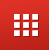Fin Shorts| February 24, 2023

# How Gold Loan EMI Calculated?

EMI (Equated Monthly Instalment) on Gold Loan is the amount of money you pay every month until the loan is fully repaid. It is calculated using the following factors:

• Loan amount: The amount of money borrowed, is the main factor that determines the EMI.
• Interest Rate: The interest rate is also an important factor that determines the EMI. The higher the interest rate, the higher the EMI.
• Loan Tenure: The duration for which you take the loan also plays a significant role in determining the EMI. A longer loan tenure means lower EMI, while a shorter loan tenure means a higher EMI.
• Compounding Frequency: Compounding frequency refers to how often interest is charged on the loan. It can be monthly, quarterly, semi-annually, or annually.
• Calculation: EMI is calculated using the formula [P x R x (1+R)^N]/[(1+R)^N-1], where P is the loan amount, R is the interest rate, and N is the number of instalments.

Gold loan is an efficient and quick way to avail funds for emergencies. By understanding how EMI is calculated, you can make an informed decision about your loan and be better prepared to repay it.

78
More